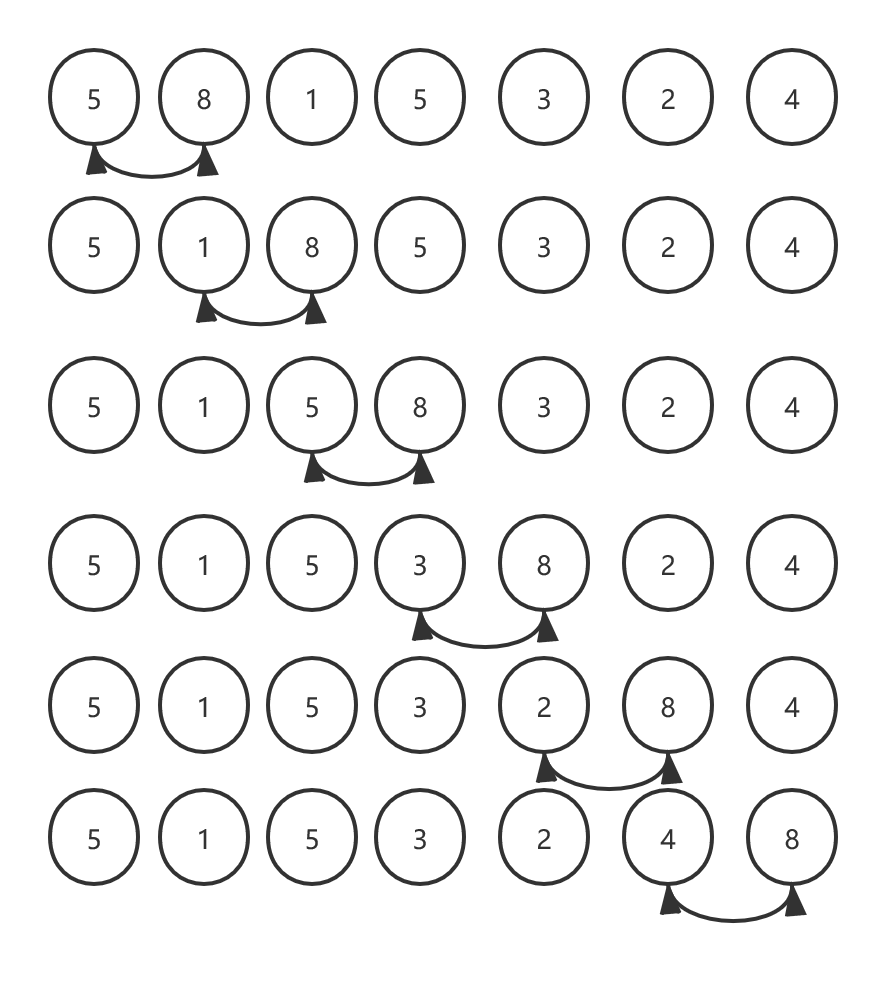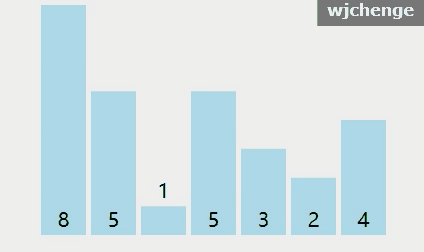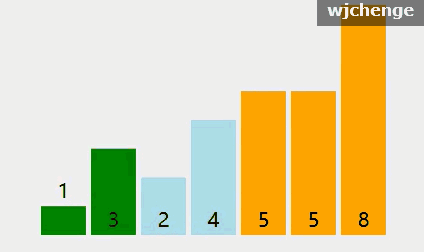# 冒泡排序wjchengepackage wjchenge.arithmetic;import java.util.Arrays;/** * 冒泡排序 * @author wjchenge */public class BubbleSort {    public static void main(String[] args) {        int[] a = new int[]{8,5,1,5,3,2,4};        System.out.println(Arrays.toString(a));        BubbleSort.bubbleSort(a);        System.out.println(Arrays.toString(a));    }    public static void bubbleSort(int[] a) {        if (a == null || a.length == 0) return;        int length = a.length;        //冒泡排序主要就是比较和交换（最后一个元素不需要比较）        for (int i = 0; i < length - 1; ++i) {            for (int j = 0; j < length - i - 1; ++j) {                if (a[j] > a[j + 1]) {                    swap(a, j, j + 1);                }            }        }    }    public static void swap(int[] a, int i, int j) {        if (a[i] != a[j]) {            a[i] = a[i] ^ a[j];            a[j] = a[i] ^ a[j];            a[i] = a[i] ^ a[j];        }    }}public static void bubbleSort(int[] a) {        if (a == null || a.length == 0) return;        int length = a.length;        //提前退出冒泡排序标识        boolean flag = true;        //冒泡排序主要就是比较和交换（最后一个元素不需要比较）        for (int i = 0; i < length - 1; ++i) {            for (int j = 0; j < length - i - 1; ++j) {                if (a[j] > a[j + 1]) {                    swap(a, j, j + 1);                    //如果存在交换则不提前退出                    flag = false;                }            }            //提前退出            if (flag) {                break;            }        }    }## 评论